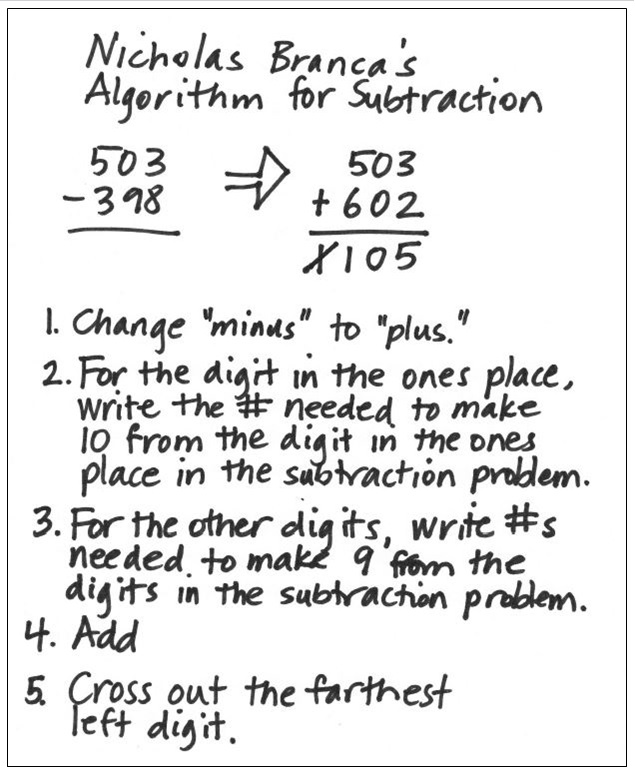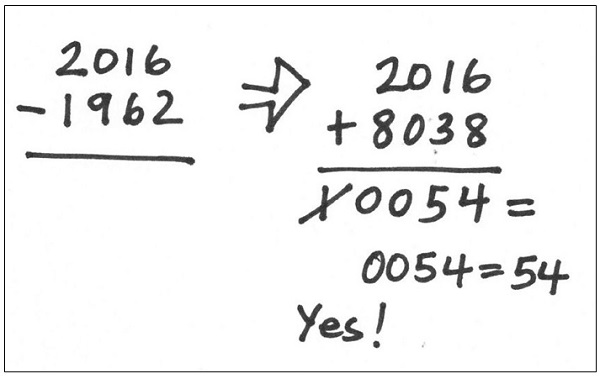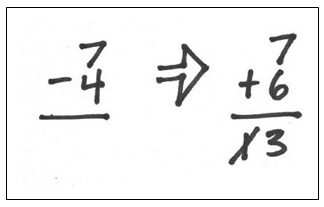OK, OK, I know that I’ve already previously posted three blogs about subtraction. But recently, when preparing to teach my friend Ruth Cossey’s elementary math methods class at Mills College, I decided to focus on subtraction as a way to talk about the balance between developing understanding and developing skills.

A Beginning Problem
As an introduction, I told the class that I began teaching in 1962 and asked them to figure out how many years I’ve been a teacher. I asked them to solve this problem in their heads, without paper and pencil. (You might want to try this first before reading what Ruth’s students reported.)

They all got the correct answer—54 years (an amazing thought to me)—but they reported several different ways of figuring.

• One student added 40 to 1962 to get 2002, and then added 14 more to get the answer of 54 years.
• Another went two years back to 1960 and figured out that 1960 was 56 years ago, and then subtracted 2 years.
• Another saw it as a subtraction problem on her mental chalkboard, solving 2016 – 1962.
• Another counted up by 10s from 1962, keeping track of the 10s on her fingers—1972, 1982, 1992, 2002, 2012—and then added on 4 more.

Some solved the problem as an addition problem while others solved it by subtracting. This helped illustrate the importance of students seeing the relationship between addition and subtraction.

So, Why Not Just Add When You Have to Subtract?
Students typically learn to add before learning to subtract, and paper-and-pencil addition doesn’t seem to pose the difficulties that subtraction does. This inspired my dear friend and colleague Nicholas Branca to come up with an algorithm for subtracting that builds on students’ strength with adding, instead of them having to learn a completely unrelated algorithm.

When subtracting, Nicholas would change the problem to an addition problem following a specific procedure. (Well, aren’t all algorithms “specific procedures”?) Below is a step-by-step explanation for using Nicholas’s method to figure out that 503 – 398 is equal to 105. [This is a problem I wrote about in an earlier blog post Why I Like Using Open Number Lines (Though I Don’t Like the Name).]To clarify: In order to change 398 in the subtraction problem to 602 in the addition problem, first look at the 8 in the ones place in the subtraction problem and think, How much more to get to 10? It’s 2, which becomes the digit in the ones place in the addition problem. Then look at the other two digits in the subtraction problem, the 3 and 9 in 398. Think for each, How much more to get to 9? That’s how I got the 6 and the 0 in the addition problem.

Say What?
Students in Ruth’s class had the same reaction that I had when Nicholas first presented this method to me: What is going on here? Here were their questions and my responses.

Q: Can you do that?
A: I just did, and got the correct answer.

Q: Will the method work for every problem?
A: Try it for 2016 – 1962 and see if you get 54. That won’t prove whether or not it works for all problems, but at least it’s a way to try the method for yourself.

Q: How come you figured out the number to add in the ones place by adding up to 10, but figured out the numbers in the other places by adding up to 9?
A: That’s how Nicholas taught me.

Q: Why did you cross out the farthest left digit?
A: Again, that’s how Nicholas taught me. He reminded me that we “cross out” when we subtract using the traditional algorithm, so why not here.

Q: WHY DOES THIS METHOD WORK?
A: Ahhh, that’s an interesting mathematical question. Let’s try and figure out why. That’s the kind of mathematical thinking we need to do with any algorithm we teach.

Does Nicholas’s Algorithm Really Work for 2016 – 1962?
Yes. Here’s how I used it to figure out that I’ve been teaching for 54 years.Why Does Nicholas’s Algorithm Work?
I decided to think about why Nicholas’s method works by considering a simpler problem: 7 – 4. To use Nicholas’s algorithm, I changed 7 – 4 to the addition problem 7 + 6 (following step #2 and figuring that I needed to add 6 to the 4 to get 10). That resulted in a sum of 13. I crossed out the 1 and got the correct answer of 3. Violà!Then I noticed that since the 1 in the sum of 13 is in the tens place, crossing it out is the same as subtracting 10.

So what I had done, mathematically, was change 7 – 4 to 7 + 6 – 10.

It seemed clearer to me if I put in parentheses: 7 – 4 = 7 + (6 – 10)

And 6 – 10 is the same as -4.

And 7 + -4 = 3.

This isn’t a proof, but it was a way for me to work my way into the problem, trying to sneak up on a proof. I’ll leave it there.

Should We Be Teaching Nicholas’s Algorithm?
No, I’m not recommending that, certainly not with the goal of building students’ proficiency with using Nicholas’s algorithm. Nicholas’s goal was to point out that not understanding his algorithm may be the same as students not understanding when taught how to regroup with the traditional algorithm. He presented the opportunity to experience what many students experience trying to learn the procedure for paper-and-pencil subtraction.

But if you think your students would benefit from trying to figure out why Nicholas’s method makes mathematical sense, go for it. Also, it might be good for students studying algebra to think about how to justify Nicholas’s algorithm algebraically. Or perhaps a more general goal might be to help students understand what an algorithm is.

And What Exactly Is an Algorithm?
An algorithm is a step-by-step procedure that provides a reliable and efficient way to compute for a broad class of problems. That’s what I wrote in a previous blog post, Another Way to Subtract .  In that post, I also address what the difference (not meant to be a pun, really) is between an algorithm and a strategy.

A Final Note
I’ve been thinking about subtraction for a long while. In addition to the two previous blog posts I’ve cited, there’s one more, Yet Another Way to Subtract. If you’re interested, check it out. Maybe I should have titled this post Yet Again Another Way to Subtract.

•Cindy Wilson says:

When I read the title to your post, my mind went immediately to the relationship between addition and subtraction. I thought the strategy would be turning the subtraction problem into a missing addend addition problem. Boy, was I wrong! I was also amazed at the student’s thinking! I, like you, would never want to try to teach that strategy, but it is just another example of how all student’s make sense of computation in different ways. Rather than discourage them and insist on their doing it our way, we should use it as an opportunity to show the structure and patterns that are found in all areas of math. I look forward to your sharing many other ways that students make sense of subtraction.

•Susan Jones says:

Looks like a teaching moment to me 🙂 It’s a pretty cool algorithm and … really, not *that* much more complicated than “borrowing.” I’m thinking of my students who’ve memorized (for subtracting something like 1000 – 324) “nines down the line, ten at the end…” and that’s not all that different from making ten in the ones place and building to 9 in the rest of them.
I love it as helping us to *not* assume that our students are Getting the Right Answer are understanding what’s going on.

•Dave Posner says:

(As I’m sure you know) Nicholas’s algorithm is based on modular arithmetic. It is the base 10 version of “2’s complement” which is the now universal representation of numbers in computer hardware. (Some systems used to use something called 1’s complement but I don’t think any do now.)

Modular arithmetic would seem to be too hard for elementary school students but maybe not? After all base 10 is based on modular arithmetic too.

If we were going to change the base 10 algorithm we teach I still think we should teach the “Trouble’s coming” method that you presented in a previous blog which simplifies approximation and according to wiki is the procedure taught in some other countries.

•Marilyn Burns says:

I’m with you on the Trouble Coming algorithm. It’s so … well … tidy.

•NathanT says:

HAHAHAHA!

I saw an e-mail which linked three blog posts regarding subtraction. I started reading in the order linked (which apparently was the order written, a couple written a year ago, and then this one more recent.)

I didn’t get more than half way through the first sentence of the second paragraph when I knew my own point (not expressed on this blog until today, presuming Marilyn approves my posts) was being made. Addition and subtraction are one in the same (just inverse). Even the very first problem example, how many years teaching starting from 1962 and going to 2016.

Again, similar to an earlier post, I just reason 38 + 16 = 54. Or in my mind 100-62 = 38, 0 + 16 = 16; 38+16 = 54 years. The sum of both sides of the 100 (2000). Again, the irony here, is as I have been saying, addition and subtraction are just one in the same.

I was a bit startled, however, to follow the rest of this post, with an “algorithm” for converting a subtraction problem to addition, 503 – 398 = 503 + 602 – 1000.

What my mathematical mind sees is that essentially to get the number to “add,” you are just figuring (calculating one digit at a time) the “difference’ between the subtracted number and a rounded number rounded up to the next even digit (in this case one thousand). Then removing that final digit.

That is an extremely funny way to do it, and yet, I get also why it works. My mind wouldn’t do it that way, but sure, if you want to create an algorithm with those steps, it makes sense. But, unless you teach the children WHY it works (e.g. algebra) … you get responses like “that’s how Nicholas taught me.”

I like how you use algebraic reasoning in your post to identify how it works. But, it still felt lacking to me. Well, at least to the way my mind works anyway. At least you did suggest not to teach students to use that “algorithm” as written. If students understood the relationship of numbers and algebraic reasoning in general their minds can create many such methods that work for them to accomplish the same goal. Hence the term “mathematical thinking.”

Again, I show my own mathematical thinking. If I were to convert the first example to addition from subtraction it would look something more like 503 – 398 = x is inverted to 398 + x = 503. 2 + 100 + 3 = 105; again the summation of the parts below 400, and above 500, and the additional 100 between them.

Why do I go at the even 100’s? because my mind more easily processes double digit (0-99) numbers.

In any case, my point is that addition and subtraction are one in the same (only inverse of each other). And students should be taught that.

•Julia says:

As each child had different understandings, each child prefers different methods in solving problems. For example, when it comes to four fundamental rules of arithmetic, some use associative strategy or some prefer to use distributive strategy. Also for this example, there are some students who thinks that subtraction to addition make it easier for them to solve problems.

“One student added 40 to 1962 to get 2002, and then added 14 more to get the answer of 54 years.
Another went two years back to 1960 and figured out that 1960 was 56 years ago, and then subtracted 2 years.
Another saw it as a subtraction problem on her mental chalkboard, solving 2016 – 1962.
Another counted up by 10s from 1962, keeping track of the 10s on her fingers—1972, 1982, 1992, 2002, 2012—and then added on 4 more. “

What children feel easier about use of subtraction is that children can make unit digits as 0 to make calculation easier.

Another astonishing part was the method of Nicholas’ Algorithm. Rather than using a subtraction, this strategy uses the other way around and teach us to get answer using by addition strategy. Even though this is very good alternative method, I personally think this is more difficult and inefficient way to get answer. However, I agree that teaching Nicholas’s Algorithm can be good opportunity to experience the strategy.

•Marilyn Burns says:

Thanks, Julia, for your thoughts. About Nicholas’ algorithm, it’s not a procedure I teach to students, but rather find it valuable to talk about with teachers as an example of what it’s like to be expected to learn a procedure and not understand why it works. If I were to use it with students in the classroom, I think that my focus would be on trying to figure out and explain why it works. I haven’t tried that yet. Thanks again for writing.

•thanh dang says:

When I first started reading your article I thought you were going to talk about how subtraction and addition relate to one another. I thought it was neat how you showed as a technique that we can use to do subtraction such as making the number you are subtracting into 10’s. When I first looked at your technique it looked confusing and I thought that you were adding 100’s to make it easier to subtract but you were making the numbers into 10s. Your technique works but it can get confusing to other children when they are learning how to do math. They might wonder where are all these numbers coming from and it could cause some confusion.

•Marilyn Burns says:

This isn’t a method I advise teaching to children, but rather something interesting for teachers to explore to think about the relationship between addition and subtraction, and also aboutthe limits of learning an algorithm without understanding. It was beneficial for me to figure out why the method worked, and to realize how hard that can be for some students.

•Jules Bonin-Ducharme says:

Saw this example for the firstime at NCSM2016. Got me thinking of the method of subtraction in computer sciences. Made a blog post about it.
https://jboninducharme.wordpress.com/2016/05/09/nicholas-brancas-algorithm/

•fnbptown.com says:

The same change method uses the fact that adding or subtracting the same number from the minuend and subtrahend does not change the answer.

•tf7.com says:

Addition requires two numbers in scientific notation to be represented using the same exponential part, so that the significand can be simply added or subtracted.